Last Update June 17, 2002 　日本語はこちら

#### NUMERICAL SPACE / SPHERES by QUALTIC

I transfigurated the sphere shape variously by POV's qualtic.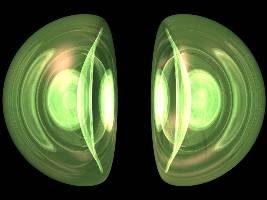Scene file : lpqcoupl.txt A COUPLE / 48k / 2002.06Scene file : lpqgourd.txt A PROCESS / 38k / 2002.06
I made these 3D images by using this function on POV's quartic.
( x^2 + y^2 + z^2 - 1 ) ( ( x - a )^2 + y^2 + z^2 - b^2 ) = c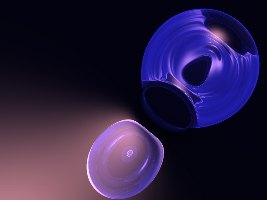Scene file : lpqpolyp.txt A POLYP / 46k / 2002.06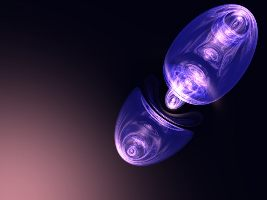Scene file : lpqseced.txt A SECESSION / 49k / 2002.06
I made these 3D images by using this function on POV's quartic.
( x^2 + ( b y )^2 + z^2 -1 ) ( ( b y ) - a ) = c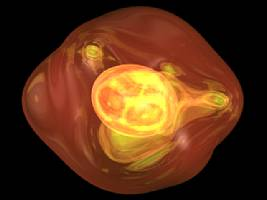Scene file : lpqcell.txt A CELL / 40k / 2002.06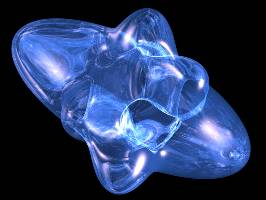Scene file : lpqspndl.txt A SPINDLE / 65k / 2002.06
I made these 3D images by using this function on POV's quartic.
( x^2 + ( a y )^2 + ( a z )^2 - 1 ) ( ( a x )^2 + y^2 + ( a z )^2 - 1 ) = cScene file : lpqring.txt A RING / 42k / 2002.06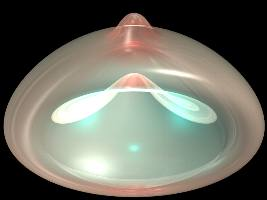Scene file : lpqdream.txt A DREAM / 30k / 2002.06
I made these 3D images by using this function on POV's quartic.
x^2 + ( a ( y + b ( x^2 + z^2 ) ) )^2 + z^2 - 1 = c

All rights reserved.